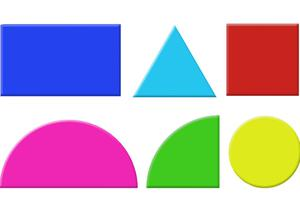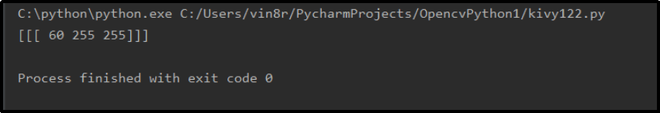# Color Identification in Images using Python – OpenCV

An open-source library in Python, OpenCV is basically used for image and video processing. Not only supported by any system, such as Windows, Linux, Mac, etc. but also it can be run in any programming language like Python, C++, Java, etc. OpenCV also allows you to identify color in images. Don’t you know how to find these colors in images?

## Color Recognition in Images

A monitor or TV screen basically generates three types of colors, i.e., red, green, and yellow. But the combination and intensities of these three colors make various colors. Thus, each color has its unique HSV color code. For finding the specified color in the given image, we need to use the lower and upper bound of that color.

Example: For finding the green color in the image, we need to specify the lower and upper HSV color code for green color as follows.

```lower=np.array([50, 100,100])
upper=np.array([70, 255, 255])```

## How to identify color in OpenCV?

Let’s try to practically implement this method. For instance, consider the below-given page source. In this source code, we are finding the green color in the shapes.jpg image. The image used in the example given belowYou can download this and save it in the same folder where you have saved the python file in which you are currently writing.

Implementation:

## Python

 `# Python program to identify ` `#color in images ` ` `  `# Importing the libraries OpenCV and numpy ` `import` `cv2 ` `import` `numpy as np ` ` `  `# Read the images ` `img ``=` `cv2.imread(``"Resources/shapes.jpg"``) ` ` `  `# Resizing the image ` `image ``=` `cv2.resize(img, (``700``, ``600``)) ` ` `  `# Convert Image to Image HSV ` `hsv ``=` `cv2.cvtColor(image, cv2.COLOR_BGR2HSV) ` ` `  `# Defining lower and upper bound HSV values ` `lower ``=` `np.array([``50``, ``100``, ``100``]) ` `upper ``=` `np.array([``70``, ``255``, ``255``]) ` ` `  `# Defining mask for detecting color ` `mask ``=` `cv2.inRange(hsv, lower, upper) ` ` `  `# Display Image and Mask ` `cv2.imshow(``"Image"``, image) ` `cv2.imshow(``"Mask"``, mask) ` ` `  `# Make python sleep for unlimited time ` `cv2.waitKey(``0``) `

Output:### Finding HSV code from RGB code

Don’t you know how to find this unique HSV color code? Just write this below-given program to find out the HSV color code from RGB color code. You can choose the RGB color code of the color you want from here. After finding the RGB code for the color you want to find, write this program to HSV code for that color.

Example: In the below source code, we have found the HSV value for the green color. The RGB value for the green color is [0, 255, 0].

## Python

 `# Python programs to find ` `# unique HSV code for color ` ` `  `# Importing the libraries openCV & numpy ` `import` `cv2 ` `import` `numpy as np ` ` `  `# Get green color ` `green ``=` `np.uint8([[[``0``, ``255``, ``0``]]]) ` ` `  `# Convert Green color to Green HSV ` `hsv_green ``=` `cv2.cvtColor(green, cv2.COLOR_BGR2HSV) ` ` `  `# Print HSV Value for Green color ` `print``(hsv_green) ` ` `  `# Make python sleep for unlimited time ` `cv2.waitKey(``0``) `

Output:Once, you have found the unique HSV code for a particular color, get the lower HSV bound and upper HSV bound of that color by following the below steps.

```lower = [h-10, 100, 100]
upper = [h+10, 255, 255]```

Example: For the green color, HSV color code is [60, 255, 255]. Hence, the lower and upper HSV bound of that color will be as follows.

```lower = [50, 100, 100]
upper = [70, 255, 255]```

Whether you're preparing for your first job interview or aiming to upskill in this ever-evolving tech landscape, GeeksforGeeks Courses are your key to success. We provide top-quality content at affordable prices, all geared towards accelerating your growth in a time-bound manner. Join the millions we've already empowered, and we're here to do the same for you. Don't miss out - check it out now!